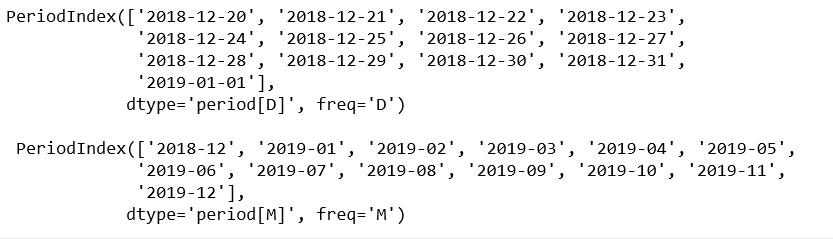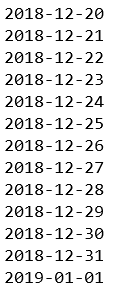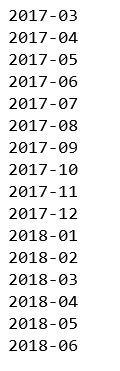# Python | pandas.period_range() method

• Last Updated : 17 Dec, 2018

Python is a great language for doing data analysis, primarily because of the fantastic ecosystem of data-centric python packages. Pandas is one of those packages and makes importing and analyzing data much easier.

`pandas.period_range()` is one of the general functions in Pandas which is used to return a fixed frequency PeriodIndex, with day (calendar) as the default frequency.

Syntax: pandas.to_numeric(arg, errors=’raise’, downcast=None)

Parameters:
start : Left bound for generating periods
end : Right bound for generating periods
periods : Number of periods to generate
freq : Frequency alias
name : Name of the resulting PeriodIndex

Returns: PeriodIndex

Code #1:

 `# importing pandas as pd ` `import` `pandas as pd ` ` `  `# period_range with freq = day ` `per1 ``=` `pd.period_range(start ``=``'2018-12-20'``, ` `              ``end ``=``'2019-01-01'``, freq ``=``'D'``) ` ` `  `# period_range with freq = month ` `per2 ``=` `pd.period_range(start ``=``'2018-12-20'``, ` `              ``end ``=``'2019-12-01'``, freq ``=``'M'``) ` ` `  `print``(per1, ``"\n\n"``, per2) `

Output:Code #2:

 `# importing pandas as pd ` `import` `pandas as pd ` ` `  `# period_range with freq = day ` `per1 ``=` `pd.period_range(start ``=``'2018-12-20'``,  ` `              ``end ``=``'2019-01-01'``, freq ``=``'D'``) ` ` `  `for` `val ``in` `per1: ` `    ``print``(val) `

Output:Code #3:

 `# importing pandas as pd ` `import` `pandas as pd ` ` `  `# Calling with pd.Period ` `per ``=` `pd.period_range(start ``=` `pd.Period(``'2017Q1'``, freq ``=``'Q'``), ` `                ``end ``=` `pd.Period(``'2018Q2'``, freq ``=``'Q'``), freq ``=``'M'``) ` ` `  `for` `val ``in` `per: ` `    ``print``(val) `

Output:My Personal Notes arrow_drop_up
Recommended Articles
Page :